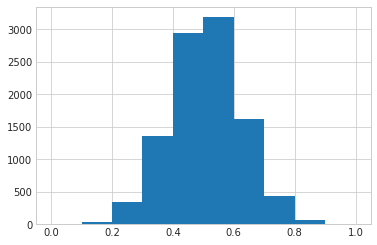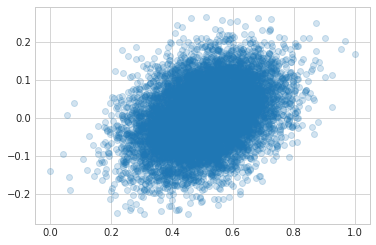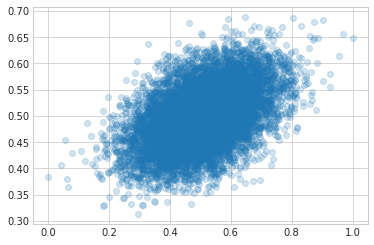# Regression of a Proportion in Python

I frequently predict proportions (e.g., proportion of year during which a customer is active). This is a regression task because the dependent variables is a float, but the dependent variable is bound between the 0 and 1. Googling around, I had a hard time finding the a good way to model this situation, so I’ve written here what I think is the most straight forward solution.

I am guessing there’s a better way to do this with MCMC, so please comment below if you know a better way.

Let’s get started by importing some libraries for making random data.

Create random regression data.

Shrink down the dependent variable so it’s bound between 0 and 1.

Make a quick plot to confirm that the data is bound between 0 and 1.All the data here is fake which worries me, but beggars can’t be choosers and this is just a quick example.

Below, I apply a plain GLM to the data. This is what you would expect if you treated this as a plain regression problem

Here’s the actual values plotted (x-axis) against the predicted values (y-axis). The model does a decent job, but check out the values on the y-axis - the linear model predicts negative values!Obviously the linear model above isn’t correctly modeling this data since it’s guessing values that are impossible.

I followed this tutorial which recommends using a GLM with a logit link and the binomial family. Checking out the statsmodels module reference, we can see the default link for the binomial family is logit.

Below I apply a GLM with a logit link and the binomial family to the data.

Here’s the actual data (x-axis) plotted against teh predicted data. You can see the fit is much better!CPython 3.6.3
IPython 6.1.0

numpy 1.13.3
matplotlib 2.0.2
sklearn 0.19.1
seaborn 0.8.0
statsmodels 0.8.0

compiler   : GCC 7.2.0
system     : Linux
release    : 4.13.0-38-generic
machine    : x86_64
processor  : x86_64
CPU cores  : 4
interpreter: 64bit